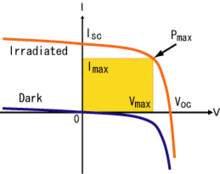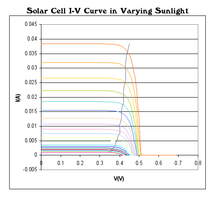# Maximum power point tracking

Maximum power point trackingCurrent-voltage characteristics of a solar cell at a particular light level, and in darkness. The area of the yellow rectangle gives the output power. Pmax denotes the maximum power point

Maximum power point tracking (MPPT) is a technique that grid tie inverters, solar battery chargers and similar devices use to get the maximum possible power from the PV array. Solar cells have a complex relationship between solar irradiation, temperature and total resistance that produces a non-linear output efficiency known as the I-V curve. It is the purpose of the MPPT system to sample the output of the cells and apply a resistance (load) to obtain maximum power for any given environmental conditions. Essentially, this defines the current that the inverter should draw from the PV in order to get the maximum possible power (since power equals voltage times current).

## I-V curveSolar cell I-V curves where a line intersects the knee of the curves where the maximum power point is located.

Photovoltaic cells have a complex relationship between their operating environment and the maximum power they can produce. The fill factor, more commonly known by its abbreviation FF, is a parameter which characterizes the non-linear electrical behavior of the solar cell. Fill factor is defined as the ratio of the maximum power from the solar cell to the product of Voc and Isc, and in tabulated data it is often used to estimate the power that a cell can provide with an optimal load under given conditions, P=FF*Voc*Isc. For most purposes, FF, Voc, and Isc are enough information to give a useful approximate model of the electrical behavior of a photovoltaic cell under typical conditions.

For any given set of operational conditions, cells usually have a single operating point where the values of the current (I) and Voltage (V) of the cell result in a maximum power output. These values correspond to a particular load resistance, which is equal to V/I as specified by Ohm's Law. The power P is given by P=V*I. A photovoltaic cell has an approximately exponential relationship between current and voltage (taking all the device physics into account, the model can become substantially more complicated though). As is well known from basic circuit theory, the power delivered from or to a device is optimized where the derivative (graphically, the slope) dI/dV of the I-V curve is equal and opposite the I/V ratio (where dP/dV=0). This is known as the maximum power point (MPP) and corresponds to the "knee" of the curve.

A load with resistance R=V/I equal to the reciprocal of this value is the load which draws maximum power from the device, and this is sometimes called the characteristic resistance of the cell. Note however that this is a dynamic quantity which changes depending on the level of illumination, as well as other factors such as temperature and the age of the cell. If the resistance is lower or higher than this value, the power drawn will be less than the maximum available, and thus the cell will not be used as efficiently as it could be. Maximum power point trackers utilize different types of control circuit or logic to search for this point and thus to allow the converter circuit to extract the maximum power available from a cell.

## Classification

There are three main types of MPPT algorithms: perturb-and-observe, incremental conductance and constant voltage. The first two methods are often referred to as hill climbing methods, because they depend on the fact that on the left side of the MPP, the curve is rising (dP/dV > 0) while on the right side of the MPP the curve is falling (dP/dV < 0).

### Perturb-and-observe (P&O) method

This method is the most common. The algorithm perturbs the operating voltage in a given direction and samples dP/dV. If dP/dV is positive, then the algorithm knows it adjusted the voltage in the direction toward the MPP. It keeps adjusting the voltage in that direction until dP/dV is negative.

P&O algorithms are easy to implement, but they sometimes result in oscillations around the MPP in steady-state operation. They also have slow response times and can even track in the wrong direction under rapidly changing atmospheric conditions.

### Incremental conductance (INC) method

This method uses the PV array's incremental conductance dI/dV to compute the sign of dP/dV. INC tracks rapidly changing irradiance conditions more accurately than the P&O method. However, like the P&O method, it can produce oscillations and be confused by rapidly changing atmospheric conditions. Another disadvantage is that its increased complexity increases computational time and slows down the sampling frequency.

### Constant voltage method

This method makes use of the fact that the ratio of maximum power point voltage to the open circuit voltage is often close to a constant value, with 0.76 being a common estimate. One problem with this method arises from the fact that it requires momentarily setting the PV array current to 0 to measure the array's open circuit voltage. The array's operating voltage is then set to (for example) 76% of this measured value. But during the time the array is disconnected, the available energy is wasted. It has also been found that while 76% of the open circuit voltage is often a very good approximation, it does not always coincide with the maximum power point. Thus this method may not give as much efficiency as others, especially if conditions are highly variable or the physical behavior of the cell deviates from expectations. Its main advantage is that it is relatively simple to implement and thus usually less expensive.

## MPPT placement

Traditional solar inverters perform MPPT for an entire array as a whole. In such systems the same current, dictated by the inverter, flows through all panels in the string. But because different panels have different IV curves, i.e. different MPPs (due to manufacturing tolerance, partial shading, etc.) this architecture means some panels will be performing below their MPP, resulting in the loss of energy.

Some companies (see power optimizer) are now placing peak power point converters into individual panels, allowing each to operate at peak efficiency despite uneven shading, soiling or electrical mismatch.

## Operation with batteries

At night, an off-grid PV power system uses batteries to supply its loads. Although the battery pack voltage when fully charged may be close to the PV array's peak power point, this is unlikely to be true at sunrise when the battery is partially discharged. Charging may begin at a voltage considerably below the array peak power point, and a MPPT can resolve this mismatch.

When the batteries in an off-grid system are full and PV production exceeds local loads, a MPPT can no longer operate the array at its peak power point as the excess power has nowhere to go. The MPPT must then shift the array operating point away from the peak power point until production exactly matches demand. (An alternative approach commonly used in spacecraft is to divert surplus PV power into a resistive load, allowing the array to operate continuously at its peak power point.)

In a grid-tied photovoltaic system, the grid is essentially a battery with near infinite capacity. The grid can always absorb surplus PV power, and it can cover shortfalls in PV production (e.g., at night). Batteries are thus needed only for protection from grid outages. The MPPT in a grid tied PV system will always operate the array at its peak power point unless the grid fails when the batteries are full and there are insufficient local loads. It would then have to back the array away from its peak power point as in the off-grid case (which it has temporarily become).

## MPPT as a motor drive

MPPTs can be designed to drive an electric motor without a storage battery. They provide significant advantages, especially when starting a motor under load. This can require a starting current that is well above the short-circuit rating of the PV panel. A MPPT can step the panel's relatively high voltage and low current down to the low voltage and high current needed to start the motor. Once the motor is running and its current requirements have dropped, the MPPT will automatically increase the voltage to normal. In this application, the MPPT can be seen as an electrical analogue to the transmission in a car; the low gears provide extra torque to the wheels until the car is up to speed.

Wikimedia Foundation. 2010.

Поможем написать реферат

### Look at other dictionaries:

• Maximum power point tracker — Un MPPT, de l anglais Maximum Power Point Tracking est un principe permettant de suivre, comme son nom l indique, le point de puissance maximale d un générateur électrique non linéaire. Les systèmes MPPT sont généralement associés avec les… …   Wikipédia en Français

• Maximum Ride — Maximum Ride: The Angel Experiment Maximum Ride: School s Out Forever Maximum Ride: Saving the World and Other Extreme Sports Maximum Ride: The Final Warning MAX: A Maximum Ride Novel Fang: A Maximum Ride Novel Angel: A Maximum Ride Novel… …   Wikipedia

• Point spread function — The point spread function (PSF) describes the response of an imaging system to a point source or point object. A related but more general term for the PSF is a system s impulse response. The PSF in many contexts can be thought of as the extended… …   Wikipedia

• Solar inverter — Internal view of a solar inverter. A solar inverter or PV inverter is a critical component in a Photovoltaic system. It performs the conversion of the variable DC output of the Photovoltaic (PV) modules into a utility frequency AC current that… …   Wikipedia

• Photovoltaic system — A photovoltaic system is a system which uses solar cells to convert light into electricity. A photovoltaic system consists of multiple components, including cells, mechanical and electrical connections and mountings and means of regulating and/or …   Wikipedia

• Solar micro-inverter — A solar micro inverter: the Enphase M190 in the process of being installed. The ground wire is attached to the lug and the panel s DC connections are attached to the cables on the lower right. The AC parallel trunk cable runs at the top (just… …   Wikipedia

• Concentrated solar power — The PS10 Solar Power Plant concentrates sunlight from a field of heliostats onto a central solar power tower. Concentrated solar power (CSP) systems use mirrors or lenses to concentrate a large area of sunlight, or solar thermal energy, onto a… …   Wikipedia

• Solar cell — A solar cell made from a monocrystalline silicon wafer …   Wikipedia

• Constant voltage method — is a type of MPPT algorithm. This method makes use of the fact that the ratio of maximum power point voltage and the open circuit voltage is 0.76. It is the simplest MPPT control method. Algorithm The operating point of the PV array is… …   Wikipedia

• Solar power satellite — A solar power satellite, or SPS or Powersat, as originally proposed would be a satellite built in high Earth orbit that uses microwave power transmission to beam solar power to a very large antenna on Earth. Advantages of placing the solar… …   Wikipedia